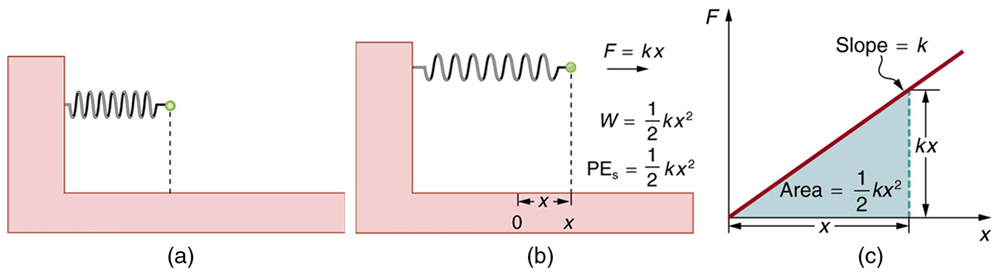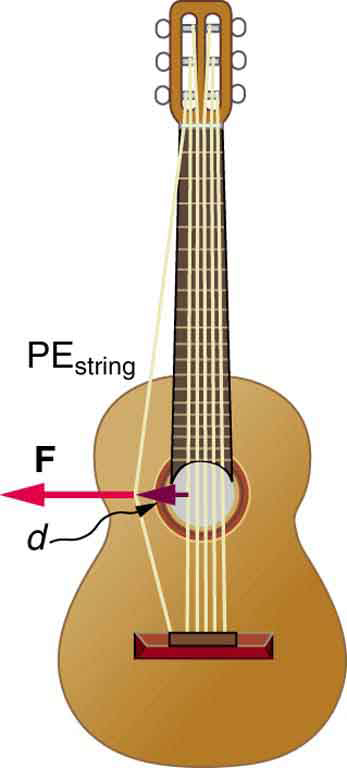# 7.4 Conservative forces and potential energy  (Page 2/8)

 Page 2 / 8
${\text{PE}}_{\text{s}}=\frac{1}{2}{\text{kx}}^{2}\text{,}$

where $k$ is the spring’s force constant and $x$ is the displacement from its undeformed position. The potential energy represents the work done on the spring and the energy stored in it as a result of stretching or compressing it a distance $x$ . The potential energy of the spring ${\text{PE}}_{s}$ does not depend on the path taken; it depends only on the stretch or squeeze $x$ in the final configuration.(a) An undeformed spring has no PE s size 12{"PE" rSub { size 8{s} } } {} stored in it. (b) The force needed to stretch (or compress) the spring a distance x size 12{x} {} has a magnitude F = kx size 12{F= ital "kx"} {} , and the work done to stretch (or compress) it is 1 2 kx 2 size 12{ { {1} over {2} } ital "kx" rSup { size 8{2} } } {} . Because the force is conservative, this work is stored as potential energy ( PE s ) size 12{ $$"PE" rSub { size 8{s} }$$ } {} in the spring, and it can be fully recovered. (c) A graph of F size 12{F} {} vs. x size 12{x} {} has a slope of k size 12{k} {} , and the area under the graph is 1 2 kx 2 size 12{ { {1} over {2} } ital "kx" rSup { size 8{2} } } {} . Thus the work done or potential energy stored is 1 2 kx 2 .

The equation ${\text{PE}}_{s}=\frac{1}{2}{\text{kx}}^{2}$ has general validity beyond the special case for which it was derived. Potential energy can be stored in any elastic medium by deforming it. Indeed, the general definition of potential energy    is energy due to position, shape, or configuration. For shape or position deformations, stored energy is ${\text{PE}}_{s}=\frac{1}{2}{\text{kx}}^{2}$ , where $k$ is the force constant of the particular system and $x$ is its deformation. Another example is seen in [link] for a guitar string.Work is done to deform the guitar string, giving it potential energy. When released, the potential energy is converted to kinetic energy and back to potential as the string oscillates back and forth. A very small fraction is dissipated as sound energy, slowly removing energy from the string.

## Conservation of mechanical energy

Let us now consider what form the work-energy theorem takes when only conservative forces are involved. This will lead us to the conservation of energy principle. The work-energy theorem states that the net work done by all forces acting on a system equals its change in kinetic energy. In equation form, this is

${W}_{\text{net}}=\frac{1}{2}{\text{mv}}^{2}-\frac{1}{2}{{\text{mv}}_{0}}^{2}=\Delta \text{KE.}$

If only conservative forces act, then

${W}_{\text{net}}={W}_{\text{c}}\text{,}$

where ${W}_{c}$ is the total work done by all conservative forces. Thus,

${W}_{\text{c}}=\text{Δ}\text{KE.}$

Now, if the conservative force, such as the gravitational force or a spring force, does work, the system loses potential energy. That is, ${W}_{\text{c}}=-\text{Δ}\text{PE}$ . Therefore,

$-\text{Δ}\text{PE}=\text{Δ}\text{KE}$

or

$\text{Δ}\text{KE}+\text{Δ}\text{PE}=0.$

This equation means that the total kinetic and potential energy is constant for any process involving only conservative forces. That is,

where i and f denote initial and final values. This equation is a form of the work-energy theorem for conservative forces; it is known as the conservation of mechanical energy    principle. Remember that this applies to the extent that all the forces are conservative, so that friction is negligible. The total kinetic plus potential energy of a system is defined to be its mechanical energy    , $\left(\text{KE}+\text{PE}\right)$ . In a system that experiences only conservative forces, there is a potential energy associated with each force, and the energy only changes form between $\text{KE}$ and the various types of $\text{PE}$ , with the total energy remaining constant.

What is the difference between a principle and a law
the law is universally proved. The principal depends on certain conditions.
Dr
it states that mass of an element deposited during electrolysis is directly proportional to the quantity of electricity discharge
Olamide
what does the speedometer of a car measure ?
Car speedometer measures the rate of change of distance per unit time.
Moses
describe how a Michelson interferometer can be used to measure the index of refraction of a gas (including air)
using the law of reflection explain how powder takes the shine off a person's nose. what is the name of the optical effect?
WILLIAM
is higher resolution of microscope using red or blue light?.explain
WILLIAM
what is dimensional consistent
Mohammed
In engineering and science, dimensional analysis is the analysis of the relationships between different physical quantities by identifying their base quantities and units of measure and tracking these dimensions as calculations or comparisons are performed
syed
can sound wave in air be polarized?
Unlike transverse waves such as electromagnetic waves, longitudinal waves such as sound waves cannot be polarized. ... Since sound waves vibrate along their direction of propagation, they cannot be polarized
Astronomy
A proton moves at 7.50×107m/s perpendicular to a magnetic field. The field causes the proton to travel in a circular path of radius 0.800 m. What is the field strength?
derived dimenionsal formula
what is the difference between mass and weight
assume that a boy was born when his father was eighteen years.if the boy is thirteen years old now, how is his father in
Isru
31yrs
Olamide
what is airflow
derivative of first differential equation
why static friction is greater than Kinetic friction
draw magnetic field pattern for two wire carrying current in the same direction
An American traveler in New Zealand carries a transformer to convert New Zealand’s standard 240 V to 120 V so that she can use some small appliances on her trip.
What is the ratio of turns in the primary and secondary coils of her transformer?
nkombo
what is energy
Yusuf
How electric lines and equipotential surface are mutually perpendicular?
The potential difference between any two points on the surface is zero that implies È.Ŕ=0, Where R is the distance between two different points &E= Electric field intensity. From which we have cos þ =0, where þ is the angle between the directions of field and distance line, as E andR are zero. Thus
sorry..E and R are non zero...

#### Get Jobilize Job Search Mobile App in your pocket Now!ByByBy OpenStaxBy OpenStaxBy OpenStaxBy Yasser IbrahimBy OpenStaxBy OpenStaxBy Stephen VoronByByBy Zarina Chocolate